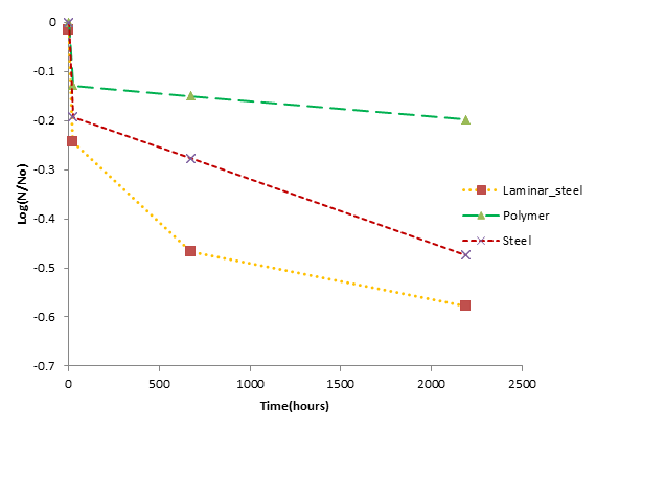Author: Jade Mitchell and Sushil B. Tamrakar

## General overview

Inactivation of vegetative microorganisms, there are several types of survival curves that described the inactivation rates and patterns(Xiong, Xie et al. 1999). The most commonly used mathematical linear model is first order exponential model. However, in many cases linear model alone cannot describe the prevailing pattern. There are several nonlinear models described by various investigators to fit the data (Coroller, Leguerinel et al. 2006). Seven different models described in various studies have been shown in Table 1.(Peleg and Cole 1998; Juneja, Eblen et al. 2001; Valdramidis, Bernaerts et al. 2005; Juneja, Huang et al. 2006).

The data from each treatment was fitted to a best-fit curve using an unpublished mathematical model fitting tool in Microsoft® Excel (Microsoft® Inc., Redmond, Washington) by Patrick Gurian at Drexel University and modified by Sushil Tamrakar (Michigan State University). The tool can be used to model bacterial survival in culture-dependent or culture-independent methods independent of the organism and the environmental conditions. The smallest absolute value of the Bayesian information criterion (BIC) was the criteria to choose the best fit model.

Table 1 Persistent models and equations

 S.N. Model Equation Curve Properties Reference 1 First order exponential decay model Ln (Nt/N0) = -kt Linear, negative slope Crane and Moore, 1986 2 Biphasic exponential decay model for 0≤t 0 nonlinear concave Gil et al. 2011 9 Gompertz-Makeham (gzm) Ln (Nt/N0)=(-k3*t -k1/k2*(exp^((k2*t)).-1)) k1, k2, k3 > 0  nonlinear concave Jodra, 2009 10 Weibull (wb) Ln (Nt/N0)=-{(t/k1)}^k2 k1= treatment time for first decimal reduction  k2=shape parameter  concave or convex Coroller et al., 2006; Mafart et al., 2002 11 Gamma (gam) Ln (Nt/N0)=(t^(k2-1) ) exp^((-t/k2)). Nonlinear concave , 12 Sigmoid-B (sB) Ln (Nt/N0)=-(k1*t^k3)/(k2+t^k3 ) Nonlinear concave or convex Peleg 2006 13 Logistic-Fermi Combination N(t)/N_0 =1/(1+exp(k1*(t-k2))) Nonlinear, concave Peleg 2006 14 Log-normal N(t)/N_0)=[1-Φ{(ln(t)-µ)/б}] Concave, nonlinear Aragao 2007. 15 Biphasic(3 parameters) if (min(t)=k3) N(t)/N_0 = exp(-k1*t[t>=k3]+k2*(t[t>=k3]-k3)) Nonlinear, concave Kamau et al. (1990) 16 Double exponential N(t)/N_0 =a exp(-k1t) + (1-a) exp(-k2t) Nonlinear, concave Peleg 2006 17 Sigmoid type A log(N(t)/N_0 )=(a_1 t)/([1+a_2 t][a_3-t]) Nonlinear, S curve Peleg 2006

The data from fomite recovery experiments in Dr. Charles Gerba’s lab were fit to the different persistent models. The best fit models are shown in Table 2 and Figure 1 .

Table 2 Best-fit models

 Organism Fomite Time(hrs) Best fit model B. anthracis Polyester 0,24,672,2190 Biphasic exponential B. anthracis Steel 0,24,672,2190 Biphasic exponential B. anthracis Laminar 0,24,672,2190 Juneja & Mark(2)Figure 1 Best fit models

## Persistence excel tool

Any persistent data could be analyzed by using attached excel spreadsheet. Read the instruction manual first and then use the excel tool accordingly.